# JP2015210323A - Lighting unit, lighting control method, and display device - Google Patents

## Info

Publication number
JP2015210323A
JP2015210323A JP2014090316A JP2014090316A JP2015210323A JP 2015210323 A JP2015210323 A JP 2015210323A JP 2014090316 A JP2014090316 A JP 2014090316A JP 2014090316 A JP2014090316 A JP 2014090316A JP 2015210323 A JP2015210323 A JP 2015210323A
Authority
JP
Japan
Prior art keywords
signal
blpwm
control signal
value
bits
Prior art date
Legal status (The legal status is an assumption and is not a legal conclusion. Google has not performed a legal analysis and makes no representation as to the accuracy of the status listed.)
Pending
Application number
JP2014090316A
Other languages
Japanese (ja)
Inventor

Naritaka Goto

Toshiyuki Nagatsuma

Akira Sakaigawa

Masaaki Kabe

Tae Kurokawa

Kojiro Ikeda

Original Assignee

Japan Display Inc
Priority date (The priority date is an assumption and is not a legal conclusion. Google has not performed a legal analysis and makes no representation as to the accuracy of the date listed.)
Filing date
Publication date
Application filed by 株式会社ジャパンディスプレイ, Japan Display Inc filed Critical 株式会社ジャパンディスプレイ
Priority to JP2014090316A priority Critical patent/JP2015210323A/en
Publication of JP2015210323A publication Critical patent/JP2015210323A/en
Pending legal-status Critical Current

• 230000000875 corresponding Effects 0 claims abstract description 33
• 230000001276 controlling effects Effects 0 claims abstract description 14
• 238000005286 illumination Methods 0 claims description 14
• 238000010191 image analysis Methods 0 claims description 5
• 238000006243 chemical reaction Methods 0 description 25
• 238000000034 methods Methods 0 description 4
• 238000005516 engineering processes Methods 0 description 3

## Images

•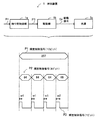•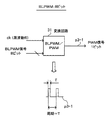•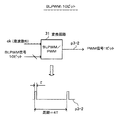•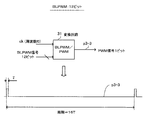•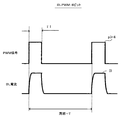•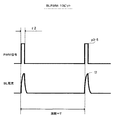•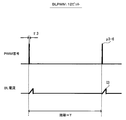•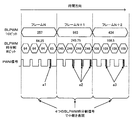•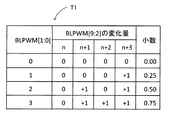•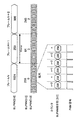•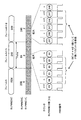•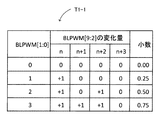•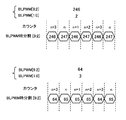•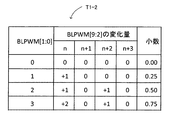••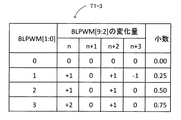•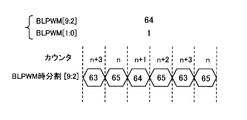•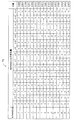•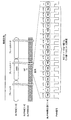•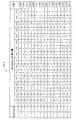•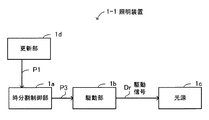••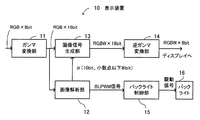•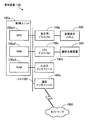•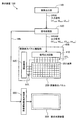## Classifications

• GPHYSICS
• G09EDUCATION; CRYPTOGRAPHY; DISPLAY; ADVERTISING; SEALS
• G09GARRANGEMENTS OR CIRCUITS FOR CONTROL OF INDICATING DEVICES USING STATIC MEANS TO PRESENT VARIABLE INFORMATION
• G09G3/00Control arrangements or circuits, of interest only in connection with visual indicators other than cathode-ray tubes
• G09G3/20Control arrangements or circuits, of interest only in connection with visual indicators other than cathode-ray tubes for presentation of an assembly of a number of characters, e.g. a page, by composing the assembly by combination of individual elements arranged in a matrix no fixed position being assigned to or needed to be assigned to the individual characters or partial characters
• G09G3/34Control arrangements or circuits, of interest only in connection with visual indicators other than cathode-ray tubes for presentation of an assembly of a number of characters, e.g. a page, by composing the assembly by combination of individual elements arranged in a matrix no fixed position being assigned to or needed to be assigned to the individual characters or partial characters by control of light from an independent source
• G09G3/3406Control of illumination source
• GPHYSICS
• G09EDUCATION; CRYPTOGRAPHY; DISPLAY; ADVERTISING; SEALS
• G09GARRANGEMENTS OR CIRCUITS FOR CONTROL OF INDICATING DEVICES USING STATIC MEANS TO PRESENT VARIABLE INFORMATION
• G09G2320/00Control of display operating conditions
• G09G2320/0626Adjustment of display parameters for control of overall brightness
• G09G2320/064Adjustment of display parameters for control of overall brightness by time modulation of the brightness of the illumination source
• GPHYSICS
• G09EDUCATION; CRYPTOGRAPHY; DISPLAY; ADVERTISING; SEALS
• G09GARRANGEMENTS OR CIRCUITS FOR CONTROL OF INDICATING DEVICES USING STATIC MEANS TO PRESENT VARIABLE INFORMATION
• G09G2320/00Control of display operating conditions
• G09G2320/0626Adjustment of display parameters for control of overall brightness
• G09G2320/0646Modulation of illumination source brightness and image signal correlated to each other

## Abstract

PROBLEM TO BE SOLVED: To suppress a reduction in image quality.SOLUTION: There is provided a lighting unit that includes: a light source; a time-division control part that performs a time division operation on a value represented by a first brightness control signal of a first bit number for controlling the brightness of the light source by a second brightness control signal with a second bit number smaller than the first bit number, so as to create a third brightness control signal with a pulse width corresponding to a value represented by the second brightness control signal; and a driving part that creates a driving signal for causing the light source to emit light on the basis of the third brightness control signal and supplies the driving signal to the light source. Also provided is a display device.

## Description

The present invention relates to an illumination device, an illumination control method, and a display device.

In recent years, liquid crystal panel displays employing the RGBW method have been developed. In this configuration, one pixel is configured by adding W (white) pixels to normal R (red), G (green), and B (blue) pixels. As a result, the brightness of the backlight for illuminating the liquid crystal panel from the back surface or the like can be lowered by the amount of improvement in the brightness of the W pixel, and the power consumption of the entire apparatus can be reduced.

On the other hand, in the display method as described above, there is a case where the image quality is lowered with the control of the luminance of the backlight. For this reason, for example, a technique is proposed in which a brightness setting value is converted into a backlight control value using a conversion table including a brightness setting value set so as to have a light emission brightness suitable for an image signal, and is supplied to the backlight. (Patent Document 1).

Alternatively, an adjustment value for adjusting the backlight luminance is calculated from the average luminance of the image for each screen and the luminance adjustment line, and a signal for driving the backlight according to the adjustment value is generated. A technique for controlling backlight luminance has been proposed (Patent Document 2).

JP 2007-322881 A JP 2010-002876 A

The present invention provides an illumination device, an illumination control method, and a display device that are improved in image quality degradation. Alternatively, an illumination device, an illumination control method, and a display device that realize high-accuracy brightness control are provided.

One embodiment of the present invention is directed to a light source and a value represented by the first luminance control signal with respect to a first luminance control signal having a first number of bits for controlling the luminance of the light source. A third luminance having a pulse width corresponding to a value represented by the second luminance control signal, time-divided by a second luminance control signal having a second bit number smaller than the first bit number. A lighting device comprising: a time division control unit that generates a control signal; and a drive unit that generates a drive signal for causing the light source to emit light based on the third luminance control signal and supplies the drive signal to the light source. .

It is a figure which shows the structural example of an illuminating device. It is a figure which shows expansion | extension of the period of a PWM signal. It is a figure which shows expansion | extension of the period of a PWM signal. It is a figure which shows expansion | extension of the period of a PWM signal. It is a figure which shows the phenomenon where the pulse width of the PWM signal per bit becomes narrow. It is a figure which shows the phenomenon where the pulse width of the PWM signal per bit becomes narrow. It is a figure which shows the phenomenon where the pulse width of the PWM signal per bit becomes narrow. It is a figure for demonstrating the concept of BLPWM time division control. It is a figure which shows the variation | change_quantity of BLPWM [9: 2]. It is a figure which shows an example of BLPWM time division control. It is a figure which shows an example of BLPWM time division control. It is a figure which shows the other version of the variation | change_quantity of BLPWM [9: 2]. It is a figure which shows BLPWM time division. It is a figure which shows the other version of the variation | change_quantity of BLPWM [9: 2]. It is a figure which shows BLPWM time division. It is a figure which shows the other version of the variation | change_quantity of BLPWM [9: 2]. It is a figure which shows BLPWM time division. It is a figure which shows the variation | change_quantity of BLPWM [11: 4]. It is a figure which shows an example of BLPWM time division control. It is a figure which shows the other version of the variation | change_quantity of BLPWM [11: 4]. It is a figure which shows the structural example of an illuminating device. It is a figure which shows the update timing of a BLPWM signal. It is a figure which shows the structural example of a display apparatus. It is a figure which shows the hardware structural example of a display apparatus. It is a figure which shows the structural example of the function with which a display apparatus is provided.

Hereinafter, embodiments of the present invention will be described with reference to the drawings.

It should be noted that the disclosure is merely an example, and those skilled in the art can easily conceive of appropriate changes while maintaining the gist of the invention are naturally included in the scope of the present invention. In addition, the drawings may be schematically represented with respect to the width, thickness, shape, and the like of each part in comparison with actual aspects for the sake of clarity of explanation, but are merely examples, and the interpretation of the present invention is not limited. It is not limited.

In the present invention and each drawing, the same reference numerals are given to the same elements as those described above with reference to the previous drawings, and the detailed description may be omitted as appropriate.

First, the present invention will be briefly described with reference to FIG. FIG. 1 is a diagram illustrating a configuration example of a lighting device. The illumination device 1 includes a light source 1c for illumination used for a time division control unit 1a, a drive unit 1b, a display panel, and the like.

The time division control unit 1a controls the luminance control signal P1 (first luminance control signal) of the first number of bits for controlling the luminance of the light source 1c, and represents a value expressed by the luminance control signal P1. Time division is performed by a luminance control signal P2 (second luminance control signal) having a second bit number smaller than the first bit number.

Then, the time division control unit 1a generates a luminance control signal P3 (third luminance control signal) having a pulse width corresponding to each value expressed by the time division luminance control signal P2.

The drive unit 1b generates a drive signal Dr for causing the light source 1c to emit light based on the luminance control signal P3, and supplies the drive signal Dr to the light source 1c.

Here, in the example of time division control shown in FIG. 1, when the first bit number is 10 bits and the second bit number is 8 bits, the value of 257 expressed by the 10-bit luminance control signal P1 is obtained. Thus, an 8-bit luminance control signal P2 having a value of 64, 64, 64, 65 divided into four is generated.

In addition, for the luminance control signal P2 having the value of 64, the pulse width (length) is w1, and for the luminance control signal P2 having the value of 65, the pulse width is w2 (> w1). A control signal P3 is generated.

Thus, in the lighting device 1, the value expressed by the luminance control signal P1 having the first number of bits is time-divided by the luminance control signal P2 having the second number of bits smaller than the first number of bits. A luminance control signal P3 having a pulse width corresponding to each value expressed by the luminance control signal P2 is generated.

And in the illuminating device 1, based on the luminance control signal P3, the drive signal Dr which light-emits the light source 1c is produced | generated, and it supplies to the light source 1c.

By such control, it becomes possible to improve the image quality deterioration, and it is possible to realize highly accurate luminance control for the backlight.

Details of the present technology will be described below. Before describing the details of the present invention, problems to be solved will be described with reference to FIGS. In an illuminating device having a backlight that illuminates the liquid crystal panel from the back or the like, the luminance of the backlight is changed according to the displayed image. The brightness of the backlight is controlled by a PWM (Pulse Width Modulated) signal.

In backlight brightness control (PWM control), a parallel bit PWM signal is usually converted into a 1-bit PWM signal, and a drive control of a backlight driver (BL (Back Light) driver) is performed using the 1-bit PWM signal. ing.

Hereinafter, the parallel bit PWM signal is referred to as a BLPWM (Back Light PWM) signal. A 1-bit PWM signal is also simply referred to as a PWM signal.

When the backlight brightness is finely controlled, the number of parallel bits of the BLPWM signal is increased and the resolution of the PWM signal is finely set. However, if the number of bits of the BLPWM signal is simply increased, the following problems occur. Occurs.

(1) In a circuit that converts a BLPWM signal having a plurality of bits into a 1-bit PWM signal, the period of the PWM signal is extended, which causes backlight flicker.

(2) Conversely, if the period of the PWM signal is maintained, the pulse width of the PWM signal per bit becomes narrow, the BL driver cannot respond to the signal with the narrow pulse width, and the luminance linearity cannot be maintained.

Hereinafter, (1) will be described with reference to FIGS. 2 to 4, and (2) will be described with reference to FIGS. 2 to 4 are diagrams showing the expansion of the period of the PWM signal.

FIG. 2 shows conversion of an 8-bit BLPWM signal to a PWM signal. A clock signal ck having a frequency f0 and an 8-bit BLPWM signal are input to the conversion circuit 31, and a 1-bit PWM signal p3-1 is output from the conversion circuit 31. Further, T represents one cycle of the PWM signal p3-1 and τ represents a pulse width of the H level of the PWM signal p3-1.

FIG. 3 shows conversion of a 10-bit BLPWM signal into a PWM signal. A clock signal ck having a frequency f0 and a 10-bit BLPWM signal are input to the conversion circuit 31, and a 1-bit PWM signal p3-2 is output from the conversion circuit 31.

At this time, when the pulse width of the H level of the PWM signal p3-2 is set to the same τ as the pulse width of the PWM signal p3-1 in FIG. 2, one cycle of the PWM signal p3-2 is 4T.

FIG. 4 shows conversion of a 12-bit BLPWM signal into a PWM signal. A clock signal ck having a frequency f0 and a 12-bit BLPWM signal are input to the conversion circuit 31, and a 1-bit PWM signal p3-3 is output from the conversion circuit 31.

At this time, if the pulse width of the H level of the PWM signal p3-3 is set to the same τ as the pulse width of the PWM signal p3-1 in FIG. 2, one cycle of the PWM signal p3-3 is 16T.

Here, the number of clocks of the clock signal ck required when generating the PWM signal from the BLPWM signal differs depending on the number of bits of the BLPWM signal.

That is, when generating a 1-bit PWM signal from an 8-bit BLPWM signal, 256 (= 2 8 ) clocks are required. When generating a 1-bit PWM signal from a 10-bit BLPWM signal, 1024 (= 2 10 ) clocks are required. Further, when a 1-bit PWM signal is generated from a 12-bit BLPWM signal, 4096 (= 2 12 ) clocks are required.

On the other hand, like the conversion circuit 31 described above, the frequency (number of clocks) of the input clock signal is fixed, the number of bits of the BLPWM signal is changed, and the pulse width of the PWM signal output from the conversion circuit 31 is changed. , The pulse width is set to the same value as the minimum number of bits of the BLPWM signal. Then, as the number of bits of the BLPWM signal increases, the cycle of the PWM signal output from the conversion circuit 31 is extended.

That is, as shown in FIGS. 2 to 4, in the conversion circuit 31, the number of bits of the BLPWM signal is increased to 8, 10, and 12 with the clock signal ck having the same frequency f0. For the 10 and 12-bit BLPWM signal, the pulse width of the PWM signal output at that time is set to the same pulse width as that of the 8-bit BLPWM signal (in the above, the H level is the same). However, the L level may be the same).

Then, when one cycle of the PWM signal output from the conversion circuit 31 when the 8-bit BLPWM signal is input is T, one cycle of the PWM signal is extended to 4T when the 10-bit BLPWM signal is input.

In addition, although one cycle of the PWM signal output from the conversion circuit 31 when the 8-bit BLPWM signal is input is T, when the 12-bit BLPWM signal is input, one cycle of the PWM signal is expanded to 16T.

In this way, if the number of bits of the BLPWM signal is simply increased to finely control the backlight brightness, the PWM signal cycle will increase (the PWM signal frequency will decrease). This causes the screen flickering of the backlight, and as a result, the image quality is lowered.

5 to 7 are diagrams showing a phenomenon in which the pulse width of the PWM signal per bit is narrowed. When the cycle of the PWM signal after conversion output is made the same for any number of parallel bits of the BLPWM signal, the pulse width of the PWM signal becomes narrow. The reason why the pulse width of the PWM signal becomes narrow will be described below.

The BL current in the figure is a current signal for setting the brightness of the backlight, and is a drive signal generated by the BL driver based on the PWM signal. Further, the brightness of the backlight is set according to the value of the BL current by supplying the BL current to an LED (Light Emitting Diode) constituting the backlight.

FIG. 5 shows the waveforms of the PWM signal p3-4 after conversion output and the BL current I1 generated by the BL driver based on the PWM signal p3-4 with respect to the 8-bit BLPWM signal.

The time required for 1-bit change of the PWM signal p3-4 with respect to the 8-bit BLPWM signal is 1/256 hours, and the period of the PWM signal p3-4 is T.

Here, if the H-level pulse width of the PWM signal p3-4 is τ1, the BL driver normally responds to the PWM signal p3-4 and has a current value necessary for setting the luminance of the backlight. It is assumed that the BL current I1 can be generated.

FIG. 6 shows the waveforms of the PWM signal p3-5 after conversion output and the BL current I2 generated by the BL driver based on the PWM signal p3-5 with respect to the 10-bit BLPWM signal.

For the 10-bit BLPWM signal, the PWM signal p3-5 has the same period T as the PWM signal p3-4 in FIG. At this time, since the time required for 1-bit change of the PWM signal p3-5 is 1/1024 hours, the pulse width of the PWM signal p3-5 is τ2 (which is narrower than the pulse width of the PWM signal p3-4 = τ1). <Τ1).

FIG. 7 shows a waveform of a PWM signal p3-6 after conversion output with respect to a 12-bit BLPWM signal and a BL current I3 generated by the BL driver based on the PWM signal p3-6.

For the 12-bit BLPWM signal, the PWM signal p3-6 also has the same period T as the PWM signal p3-4 in FIG. At this time, since the time required for 1-bit change of the PWM signal p3-6 is 1/496 hours, the pulse width of the PWM signal p3-6 is equal to the pulse width of the PWM signal p3-4 = τ1 and the PWM signal p3−3. The pulse width of 5 is τ3 (<τ2 <τ1) narrower than τ2.

Here, the H level pulse width = τ2 of the PWM signal p3-5 shown in FIG. 6 and the H level pulse width = τ3 of the PWM signal p3-6 shown in FIG. 7 can be responded by the BL driver. The pulse width is a predetermined value or less.

In this case, even if the PWM signal p3-5 having the pulse width = τ2 is input to the BL driver, the BL driver cannot normally respond to the PWM signal p3-5. The current cannot be generated.

Similarly, even if the PWM signal p3-6 having the pulse width = τ3 is input to the BL driver, the BL driver cannot normally respond to the PWM signal p3-6, so that the BL necessary for driving the backlight is required. The current cannot be generated.

This is because when the pulse width of the PWM signal input to the BL driver is equal to or smaller than a predetermined value, the PWM signal rises before the BL current rise is sufficiently secured when the BL current rises following the rise of the PWM signal. This is because the information represented by the PWM signal cannot be reflected in the current amount of the BL current.

As described above, when the number of bits of the BLPWM signal is increased to finely control the luminance of the backlight, the pulse width of the PWM signal per bit is narrowed to a predetermined value or less. When the pulse width of the PWM signal is narrowed, the BL driver cannot respond normally and the luminance linearity cannot be maintained. Further, if the luminance linearity cannot be maintained, as a result, the image quality is lowered.

The present technology has been made in view of such a point, and realizes high-precision luminance control of a backlight and performs illumination control with the aim of improving image quality degradation.

Next, the illumination device 1 of the present technology will be described in detail. In the time division control unit 1a of the lighting device 1, even if the number of bits of the BLPWM signal increases, the resolution of the PWM signal remains unchanged, and instead, the PWM signal is changed in the time direction to increase the bit number of the BLPWM signal. (Hereinafter, the control performed by the time division control unit 1a is also referred to as BLPWM time division control).

FIG. 8 is a diagram for explaining the concept of BLPWM time-division control. The BLPWM signal is a 10-bit parallel signal. The “BLPWM 10 bit” in the figure corresponds to the luminance control signal P1 in FIG.

Further, “BLPWM time division 8 bits” in the figure corresponds to the luminance control signal P2 in FIG. Further, the “PWM signal” in the figure corresponds to the luminance control signal P3 in FIG.

On the other hand, the value (luminance gradation value) expressed by the 10-bit BLPWM signal in frame N is 257, the value expressed by the 10-bit BLPWM signal in frame N + 1 is 983, and the 10-bit BLPWM signal in frame N + 2 Assume that the value to be expressed is 434.

Consider a case in which a 10-bit BLPWM signal is converted into, for example, an 8-bit BLPWM signal as BLPWM time-division control for a frame represented by such a 10-bit BLPWM signal.

In this case, the lower 2 bits of the 10 bits are truncated, which corresponds to division by 2 2 in the bit shift operation. For frame N, 257 is divided by 2 2 to give 64.25 (= 257/4). 64.25 includes an integer part 64 that can be represented by 8 bits and a fractional part 0.25.

When a fractional part is generated as described above, a 10-bit BLPWM signal is expressed by changing at least one integer part of the 8-bit BLPWM signal. For example, in the time domain of frame N, 257 of the 10-bit BLPWM signal is time-divided into 8-bit data of 64, 64, 64, and 65 of the 8-bit BLPWM signal.

The average value of the 8-bit BLPWM signal at this time is (64 + 64 + 64 + 65) /4=64.25, and the decimal is expressed by four 64-, 64-, 64-, and 64-bit 8-bit BLPWM time-division signals. Yes.

Then, a 1-bit PWM signal having a pulse width corresponding to each value of 64 and 65 is generated. A hatched portion a1 in the figure represents a 1-bit increment of a value of 65 obtained by adding 1 to 64.

Note that the value of 8-bit data of 65 is located at the fourth position from the beginning of the time zone of frame N in FIG. 8, but may be located at any position in the time zone of frame N. However, for example, if it is positioned at the fourth position in the time slot of frame N, 65 is fixedly positioned at the fourth position in the time slot of frame N at the time of frame switching.

On the other hand, for frame N + 1, 983 is divided by 2 2 to obtain 245.75 (= 983/4). 245.75 includes an integer part 245 that can be represented by 8 bits and a fractional part 0.75.

Accordingly, 983 of the 10-bit BLPWM signal is time-divided into 8-bit data 245, 246, 246 and 246 of the 8-bit BLPWM signal in the time zone of frame N + 1.

The average value of the 8-bit BLPWM signal at this time is (245 + 246 + 246 + 246) /4=245.75, and the decimals are expressed by four 8-bit BLPWM time-division signals of 245, 246, 246, and 246, respectively. Yes.

Then, a 1-bit PWM signal having a pulse width corresponding to each value of 245 and 246 is generated. A hatched portion a2 in the figure represents a 1-bit increment of a value of 246 obtained by adding 1 to 245.

Note that the 8-bit data value of 246 is located at the second, third, and fourth positions from the beginning of the time slot of frame N + 1 in FIG. 8, but may be at any position in the time slot of frame N + 1. . However, for example, if it is positioned at the second, third, and fourth positions in the time slot of frame N + 1, 246 is fixedly placed at the second, third, and fourth positions in the time slot of frame N + 1 when switching frames. To.

Similarly, for frame N + 2, 434 is divided by 2 2 to obtain 108.5 (= 434/4). 108.5 includes an integer part 108 which can be expressed by 8 bits and a decimal point 0.5 which is a fractional part.

Therefore, the 10-bit BLPWM signal 434 is time-divided into 8-bit BLPWM signals 108, 109, 108, and 109, respectively, in the time period of the frame N + 2, and is expressed.

The average value of the 8-bit BLPWM signal at this time is (108 + 109 + 108 + 109) /4=108.5, and the decimal is expressed by four 8-bit BLPWM time-division signals of 108, 109, 108, and 109, respectively. Yes.

Then, a 1-bit PWM signal having a pulse width corresponding to the values 108 and 109 is generated. A hatched portion a3 in the figure represents a 1-bit increment of a value of 109 obtained by adding 1 to 108.

The 8-bit data value 109 is located at the second and fourth positions from the beginning of the time slot of frame N + 2 in FIG. 8, but may be at any position in the time slot of frame N + 2. However, for example, if it is positioned at the second and fourth positions in the time slot of the frame N + 2, 109 is fixedly placed at the second and fourth positions in the time slot of the frame N + 2 at the time of frame switching.

As described above, the time division control unit 1a of the lighting device 1 has a predetermined time of the BLPWM signal when the number of parallel bits of the BLPWM signal is K bits and the number of bits of the BLPWM time division signal is L (<K). 2 KL divided regions are generated from a region (for example, one frame time region). If K = 10 and L = 8, 4 (= 2 10-8 ) divided areas are generated.

Then, the luminance gradation value expressed in K bits is divided by 2 KL (expressed in 10 bits. For example, 257 is divided by 2 10-8 ).

An integer value that can be expressed by L bits is assigned to 2 KL divided regions. In this case, the average value obtained by dividing the sum of the values assigned to the 2 KL divided areas by 2 KL is assigned so as to be the value of the division result. The fractional part is assigned to one of the divided areas. For example, if the sum of 64, 64, 64, and 65 is gradually subtracted by 2 10-8 , it becomes 257/2 10-8 , so that 64, 64, 64, and 65 are assigned to the four divided areas. become.

In FIG. 8 described above, as BLPWM time division control for each frame, for example, a 10-bit BLPWM signal is time-divided into 8-bit data in the time direction, and the 8-bit data of the BLPWM time-division signal generated at that time is respectively Is converted into a 1-bit PWM signal.

Thereby, it is possible to execute high-precision brightness control. Further, when the BLPWM signal is converted into the PWM signal, even if the number of parallel bits of the BLPWM signal is different, one cycle of the PWM signal can be maintained to be the same, and luminance flicker can be suppressed.

Further, even if one cycle of the PWM signal is maintained, the change width of the PWM signal per bit is the same, so that the BL driver corresponding to the drive unit 1b in FIG. It becomes possible to ensure linearity.

Next, a case where a 10-bit BLPWM signal is expressed by an 8-bit BLPWM time division signal will be described in more detail. Hereinafter, the bit representation is represented by [MSB: LSB]. MSB (Most Significant Bit) is the most significant bit, and LSB (Least Significant Bit) is the least significant bit.

For example, the A signal [9: 0] is a 10-bit signal in which the least significant bit is 0 bit, the most significant bit is 9 bits, and the 0th to 9th bits are in total. Represents that.

For example, the B signal [9: 2] means that the B signal has 2 bits as the least significant bit and 9 bits as the most significant bit, and is a signal of 8 bits in total from the 2nd bit to the 9th bit. It represents that.

In the following description, the 10-bit parallel BLPWM signal is expressed as BLPWM [9: 0]. Further, an 8-bit integer part from the second bit to the ninth bit of the BLPWM signal is expressed as BLPWM [9: 2].

Furthermore, the 2-bit decimal part from the 0th bit to the 1st bit of the BLPWM signal is expressed as BLPWM [1: 0]. Further, the 8-bit BLPWM time division signal is expressed as BLPWM time division [9: 2].

Note that the 2 bits of the decimal part are 0.00 (10) 00 (2) , 0.25 (10) 01 (2) , 0.5 (10) 10 (2) , 0.75 (10 ) Can correspond to 11 (2) , respectively. Therefore, the decimal number 0.00 is expressed as BLPWM [1: 0] = 0 (00 (2) → 0).

The decimal number 0.25 is expressed as BLPWM [1: 0] = 1 (01 (2) → 1). Similarly, the decimal 0.50 is expressed as BLPWM [1: 0] = 2 (10 (2) → 2), and the decimal 0.75 is expressed as BLPWM [1: 0] = 3 (11 (2 )3).

FIG. 9 is a diagram showing the amount of change in BLPWM [9: 2]. The table T1 includes items of BLPWM [1: 0], BLPWM [9: 2] change amount, and decimal. The amount of change in BLPWM [9: 2] is divided into four time regions, n, n + 1, n + 2, and n + 3, and n, n + 1, n + 2, and n + 3 correspond to the count value of the counter.

Also, within one frame, this counter performs cyclic counting in the order of n → n + 1 → n + 2 → n + 3 → n → n + 1 →.

In the table T1, BLPWM [1: 0] = 0 represents a decimal number 0.00, and the counts n, n + 1, n + 2, and n + 3 in the variation amount of BLPWM [9: 2] are all “0”. Note that “0” means that the same value represented by 8-bit data from 2 bits to 9 bits of BLPWM [9: 2] is used.

For example, if the value expressed by BLPWM [9: 0] is 256, 256/4 = 64 and no decimals are included. Therefore, all the time domain values of the counts n, n + 1, n + 2, and n + 3 in one frame can be expressed by integers (= 64) of 8-bit data. In this case, the counts n, n + 1, n + 2, and n + 3 are all It becomes “0”.

BLPWM [1: 0] = 1 represents a decimal number of 0.25, and any one of the counts n, n + 1, n + 2, n + 3 in the amount of change in BLPWM [9: 2] is “+1”, and the rest Three become “0”.

Note that “+1” means a decimal representation of 2-bit data from 0 to 1 bit of BLPWM [1: 0].

For example, if the value expressed by BLPWM [9: 0] is 257, 257/4 = 64.25, which includes the decimal number 0.25. 64.25 can be represented by the average of 64, 64, 64, 65 (= 64 + 1), so three of the four time domains represent “0” to represent 64, and 1 of the four time domains One becomes “+1” and expresses 65.

In the example of FIG. 9, when BLPWM [1: 0] = 1, the three time regions of counts n, n + 1, and n + 2 are set to “0”, and one time region of count n + 3 is set to “1”. ing.

Further, BLPWM [1: 0] = 2 represents a decimal number 0.50, and any two of the counts n, n + 1, n + 2, n + 3 in the amount of change in BLPWM [9: 2] are “+1”, and the rest Two become “0”.

For example, if the value expressed by BLPWM [9: 0] is 434, 434/4 = 108.5, which includes the decimal number 0.5. Since 108.5 can be represented by the average of 108, 108, 109 (= 108 + 1), 109 (= 108 + 1), two of the four time domains represent “0” to represent 108 and represent four time domains. Two of these become “+1” to represent 109.

In the example of FIG. 9, when BLPWM [1: 0] = 2, the two time regions of count n and n + 2 are set to “0”, and the two time regions of count n + 1 and n + 3 are set to “+1”. ing.

Furthermore, BLPWM [1: 0] = 3 represents a decimal number 0.75, and any three of the counts n, n + 1, n + 2, n + 3 in the amount of change in BLPWM [9: 2] are “+1”. The remaining one is “0”.

For example, if the value expressed by BLPWM [9: 0] is 983, 983/4 = 245.75, which includes the decimal number 0.75. 245.75 can be expressed as an average of 245, 246 (= 245 + 1), 246 (= 245 + 1), and 246 (= 245 + 1), so that one of the four time domains becomes “0” to express 245, Three of the four time domains become “+1” to represent 246.

In the example of FIG. 9, when BLPWM [1: 0] = 3, one time region of count n is set to “0”, and three time regions of counts n + 1, n + 2, and n + 3 are set to “1”. ing. The other version of the change amount will be described later.

10 and 11 are diagrams illustrating an example of BLPWM time division control. An example of 10-bit → 8-bit BLPWM time division control is shown. The value expressed by BLPWM [9: 0] in frame N is 1024, the value expressed by BLPWM [9: 0] in frame N + 1 is 259, and the value expressed by BLPWM [9: 0] in frame N + 2 is 986. And

Since frame N is 256 (= 1024/4), BLPWM [9: 2] = 256, and BLPWM [1: 0] = 0 (corresponding to decimal 0.00).

For frame N + 1, since it is 64.75 (= 259/4), BLPWM [9: 2] = 64 and BLPWM [1: 0] = 3 (corresponding to the decimal number 0.75).

Furthermore, since the frame N + 2 is 246.5 (= 986/4), BLPWM [9: 2] = 246 and BLPWM [1: 0] = 2 (corresponding to the decimal 0.50).

Looking at BLPWM time division [9: 2], in FIG. 10, in frame N, BLPWM [1: 0] = 0, so that the time domain of counts n, n + 1, n + 2, and n + 3 are all “0” based on table T1. ".

Therefore, BLPWM time division [9: 2] of frame N is all 8-bit data of 256 in the time domain of counts n, n + 1, n + 2, and n + 3. Then, a 1-bit PWM signal having a pulse width corresponding to a value of 256 is generated.

On the other hand, in FIG. 11, since BLPWM [1: 0] = 3 in frame N + 1, the time domain of count n is “0” and the time domain of counts n + 1, n + 2, and n + 3 is “+1” based on table T1. .

Therefore, in the BLPWM time division [9: 2] of the frame N + 1, the time domain value of the count n is 64, and the time domain of the counts n + 1, n + 2, and n + 3 is 65. Then, a 1-bit PWM signal having a pulse width corresponding to each value of 64 and 65 is generated.

Further, in FIG. 11, in frame N + 2, BLPWM [1: 0] = 2, so that the time domain of count n and n + 2 is “0” and the time domain of count n + 1 and n + 3 is “+1” based on table T1. .

Therefore, in the BLPWM time division [9: 2] of the frame N + 2, the time domain value of the count n and n + 2 is 246, and the time domain of the count n + 1 and n + 3 is 247. Then, a 1-bit PWM signal having a pulse width corresponding to each value of 246 and 247 is generated.

By performing BLPWM time division control as described above, it is possible to maintain the minimum pulse width of the PWM signal to which the BL driver can normally respond, and also to maintain the period of the PWM signal.

In the table T1 shown in FIG. 9, when BLPWM [1: 0] = 1, the time region of the count n + 3 is set to “+1”. Further, when PWM [1: 0] = 2, the time region of count n + 1, n + 3 is “+1”, and when BLPWM [1: 0] = 3, the time region of count n + 1, n + 2, n + 3 is “+1”. It is said.

The table T1 is an example, and the positions where the change amount is “+1” are set in a distributed manner, and the set positions can be arbitrarily changed.

Next, another version of the amount of change in BLPWM [9: 2] will be described. FIG. 12 is a diagram showing another version of the amount of change in BLPWM [9: 2]. A table T1-1 in FIG. 12 shows different versions of the set location set to “+1”.

In the representation of the fractional part of the table T1-1, when BLPWM [1: 0] = 1, the time region of the count n is “+1”, and when BLPWM [1: 0] = 2, the time of the count n and n + 2 The area is set to “+1”. Further, when BLPWM [1: 0] = 3, the time regions of the counts n, n + 1, and n + 2 are set to “+1”.

FIG. 13 shows BLPWM time division. BLPWM time division [9: 2] = 246, BLPWM [1: 0] = 2 corresponding to table T1-1, BLPWM time division [9: 2] = 64, BLPWM [1: 0] = 3 Indicates the case.

When BLPWM [9: 2] = 246 and BLPWM [1: 0] = 2, in the table T1-1, the time region of the count n and n + 2 is “+1”, and the time region of the count n + 1 and n + 3 is “0”. It has become.

Accordingly, in this BLPWM time division [9: 2], 247 is located in the time region of count n, n + 2, and 246 is located in the time region of count n + 1, n + 3.

Further, when BLPWM [9: 2] = 64 and BLPWM [1: 0] = 3, in the table T1-1, the time region of the count n, n + 1, n + 2 is “+1”, and the time region of the count n + 3 is “ 0 ”.

Therefore, in this BLPWM time division [9: 2], 65 (= 64 + 1) is located in the time domain of counts n, n + 1, and n + 2, and 64 (= 64 + 0) is located in the time domain of count n + 3. Become.

FIG. 14 is a diagram showing another version of the amount of change in BLPWM [9: 2]. In the table T1 of FIG. 9, the change amount of BLPWM [9: 2] is “+1”, but in the table T1-2 of FIG. 14, in addition to “+1”, a change amount of “+2” is also set. The fractional part is expressed.

In the expression of the fractional part of the table T1-2, when BLPWM [1: 0] = 1, the time region of the count n is “+1”, and when BLPWM [1: 0] = 2, the time of the count n and n + 2 The area is set to “+1”. When BLPWM [1: 0] = 3, the time region of count n is “+2”, and the time region of count n + 2 is “+1”.

FIG. 15 shows BLPWM time division. The case where BLPWM time division [9: 2] = 64 and BLPWM [1: 0] = 3 corresponding to the table T1-2 is shown.

When BLPWM [9: 2] = 64 and BLPWM [1: 0] = 3, in the table T1-2, the time region of count n is “+2”, the time region of count n + 1, n + 3 is “0”, and count n + 2. Is set to “+1”.

Therefore, in this BLPWM time division [9: 2], 66 (= 64 + 2) is located in the time region of count n, 64 (= 64 + 0) is located in the time region of count n + 1, n + 3, and the count n + 2 65 (= 64 + 1) is located in the time domain.

FIG. 16 is a diagram showing another version of the amount of change in BLPWM [9: 2]. In the table T1 of FIG. 9, the change amount of BLPWM [9: 2] is “+1”, but in the table T1-3 of FIG. 16, in addition to “+1”, the change amount of “+2” is further increased. The amount of change of “−1” is also set to express the fractional part.

In the expression of the fractional part of the table T1-3, when BLPWM [1: 0] = 1, the time region of the count n and n + 2 is “+1”, and the time region of the count n + 3 is “−1”. When BLPWM [1: 0] = 2, the time region of the count n and n + 2 is “+1”. When BLPWM [1: 0] = 3, the time region of count n is “+2”, and the time region of count n + 2 is “+1”.

FIG. 17 shows BLPWM time division. The case of BLPWM time division [9: 2] = 64 and BLPWM [1: 0] = 1 corresponding to the table T1-3 is shown.

When BLPWM [9: 2] = 64 and BLPWM [1: 0] = 1, in the table T1-3, the time region of the count n and n + 2 is “+1”, and the time region of the count n + 1 is “0”. The time region of the count n + 3 is “−1”.

Therefore, in this BLPWM time division [9: 2], 65 (= 64 + 1) is located in the time region of count n, n + 2, 64 (= 64 + 0) is located in the time region of count n + 1, and the count n + 3 63 (= 64-1) is located in the time domain. Note that the above-described change amount setting is an example, and it is possible to set a change amount of a version other than the above.

Next, as another example of BLPWM time division control, a case where a 12-bit BLPWM signal is expressed by an 8-bit BLPWM time division signal will be described. The BLPWM signal is assumed to be 12-bit parallel. Further, it is assumed that the value expressed by the 12-bit BLPWM signal in the frame N is 3163.

Consider a case where a 12-bit BLPWM signal is converted into, for example, an 8-bit BLPWM signal as BLPWM time-division control for a frame expressed by such a 12-bit BLPWM signal.

In this case, the lower 4 bits of 12 bits are rounded down, which corresponds to division by 2 4 in the bit shift operation, so that for frame N, 3163 is divided by 2 4 and 197.6875 (= 3163). / 16). 196.875 includes an integer part 197 that can be represented by 8 bits and a fractional part 0.6875.

Therefore, in the time domain of frame N, 3163 of the 12-bit BLPWM signal is time-divided into 5 values of 197 of the 8-bit BLPWM signal and 11 values of 198 of the 8-bit BLPWM signal.

The average value of the 8-bit BLPWM signal at this time is (197 × 5 + 198 × 11) /16=1977.6875, and the 5-bit BLPWM time-division control signal of 5 197 and 11 198, respectively. Represents a decimal.

FIG. 18 is a diagram illustrating the amount of change in BLPWM [11: 4]. The table T2 includes items of BLPWM [3: 0], BLPWM [11: 4] change amounts, and decimal numbers.

The amount of change in BLPWM [11: 4] is divided into 16 time regions of n, n + 1,..., N + 14, n + 15, and n, n + 1,..., N + 14, n + 15 are counters. It corresponds to the count value.

Further, it is assumed that this counter performs a cyclic count in the order of n → n + 1 →... → n + 14 → n + 15 → n → n + 1 →.

As an expression of the fractional part of the table T2, for example, if BLPWM [3: 0] = 1, it represents a decimal number 0.0625 and counts n, n + 1,... In the amount of change in BLPWM [11: 4]. , N + 14, or n + 15 is “+1”, and the remaining 15 are “0”.

Further, for example, if BLPWM [3: 0] = 11, it represents a decimal number 0.6875, and any one of counts n, n + 1,..., N + 14, n + 15 in the amount of change in BLPWM [11: 4]. Eleven are "+1" and the remaining five are "0".

The other BLPWM [3: 0] are set in the same way of thinking, and the way of viewing the table has been described above, and the description thereof will be omitted.

FIG. 19 is a diagram illustrating an example of BLPWM time-division control. An example of 12-bit to 8-bit BLPWM time division control is shown. A value expressed by BLPWM [11: 0] in the frame N + 1 is 3163.

Since the frame N + 1 is 197.6875 (= 3163/16), BLPWM [11: 4] = 197 and BLPWM [3: 0] = 11 (corresponding to the decimal number 0.6875).

In frame N + 1, since BLPWM [3: 0] = 11, the time domain of counts n, n + 3, n + 6, n + 9, and n + 12 is “0” based on table T2, and counts n + 1, n + 2, n + 4, n + 5, n + 7, n + 8, The time domain of n + 10, n + 11, n + 13, n + 14, and n + 15 is “+1”.

Therefore, BLPWM time division [11: 4] of frame N + 1 has a time domain of counts n, n + 3, n + 6, n + 9, and n + 12 is 197 (= 197 + 0), counts n + 1, n + 2, n + 4, n + 5, n + 7, n + 8, n + 10, The time domain of n + 11, n + 13, n + 14, and n + 15 is 198 (= 197 + 1).

Then, a 1-bit PWM signal having a pulse width corresponding to each value of 197 and 198 is generated.

FIG. 20 is a diagram showing another version of the amount of change in BLPWM [11: 4]. In table T2 in FIG. 18, the change amount of BLPWM [11: 4] is “+1”, but in table T2-1 in FIG. 20, in addition to “+1”, a change amount of “+2” is also set. The fractional part is expressed. In addition, since the way of looking at the table has been described in detail, the description is omitted.

Next, another embodiment of the lighting device 1 will be described. FIG. 21 is a diagram illustrating a configuration example of a lighting device. The illumination device 1-1 includes a time division control unit 1a, a drive unit 1b, a light source 1c, and an update unit 1d. The description of the same components as those in FIG. 1 is omitted.

The updating unit 1d updates the luminance gradation value expressed by the first number of bits with respect to the luminance control signal P1 having the first number of bits. In this case, the value of the luminance gradation is updated at the timing when the luminance control signal P3 is switched from the first period to the second period.

FIG. 22 is a diagram showing the update timing of the BLPWM signal. BLPWM [9: 0] has 10-bit data representation values of 259, 986, and 1020.

In this way, the brightness gradation value of BLPWM [9: 0] is updated, but the BLPWM [9: 0] is converted to BLPWM time division [9: 2] and BLPWM time division [9: 2]. In the generation from the PWM signal to the PWM signal, the brightness gradation value of BLPWM [9: 2] is updated at the timing of switching the period of the PWM signal.

For example, the luminance gradation value of BLPWM [9: 0] is updated from 259 to 986 at the switching timing from the period F1 of the duty Dt1 of the PWM signal to the period F2 of the duty Dt2.

In addition, the luminance gradation value of BLPWM [9: 0] is updated from 986 to 1020 at the switching timing of the cycle D2 of the PWM signal from the cycle F2 of the duty Dt2 to the cycle F3 of the duty Dt3. Since a processing delay occurs, a time lag occurs between the break of the PWM signal cycle and the update timing of BLPWM [9: 0]. FIG. 22 shows an ideal state for easy understanding. Is)

By performing such control, the luminance gradation value of the PWM signal is updated without destroying one cycle of a certain duty of the PWM signal, so that it is possible to further reduce the luminance flicker of the backlight. Become.

Next, the structure of the display device including the function of the lighting device of the present invention will be described. FIG. 23 is a diagram illustrating a configuration example of a display device. The display circuit 10 includes a gamma (γ) conversion unit 11, an image analysis unit 12, an image signal generation unit 13, an inverse gamma (1 / γ) conversion unit 14, a backlight control unit 15, and a backlight 16.

The functions of the time division control unit 1a and the drive unit 1b in FIG. 1 and the function of the update unit 1d in FIG. 21 are included in the backlight control unit 15. 1 corresponds to the backlight 16.

For example, each of R (first subpixel), G (second subpixel), and B (third subpixel) gamma-converts an 8-bit input RGB signal, A 16-bit RGB signal (first image signal) is output.

When receiving the RGB signal output from the gamma conversion unit 11, the image analysis unit 12 calculates an expansion coefficient α (for example, 10 bits, 8 bits after the decimal point), and further performs BLPWM for controlling the luminance of the backlight 16. A signal (first luminance control signal) is generated.

The image signal generation unit 13 generates a W (fourth subpixel) signal based on the expansion coefficient α, and each of R, G, B, and W is, for example, a 16-bit RGBW signal (second image signal). Is output.

The inverse gamma conversion unit 14 performs inverse gamma conversion on the RGBW signal output from the image signal generation unit 13 and outputs, for example, an 8-bit RGBW signal for each of R, G, B, and W to the display side.

The backlight control unit 15 controls the luminance of the backlight 16 based on the BLPWM signal output from the image analysis unit 12.

That is, the backlight control unit 15 sets a value represented by the BLPWM signal to a value smaller than the first bit number for the BLPWM signal having the first bit number for controlling the luminance of the backlight 16. A PWM signal (third luminance control signal) having a pulse width corresponding to a value represented by the BLPWM time-division signal is time-divided by a BLPWM time-division signal (second luminance control signal) having a bit number of 2. Generate.

Then, the backlight control unit 15 generates a drive signal for causing the backlight 16 to emit light based on the PWM signal, and supplies the drive signal to the backlight 16.

Next, a hardware configuration example of the display device will be described. FIG. 24 is a diagram illustrating a hardware configuration example of the display device.

The display device 100 includes a control unit 100a, a display driver IC (Integrated Circuit) 100b, an LED driver IC 100c, an input / output interface 100d, and a communication interface 100e, which are connected to each other via a bus 100f so that signals can be input and output. ing. Further, the display device 100 includes an image display panel 200 and a planar light source device 300.

The control unit 100a includes a CPU (Central Processing Unit) 100a1, and the CPU 100a1 controls the entire apparatus. Such a control unit 100a further includes a RAM (Random Access Memory) 100a2 and a ROM (Read Only Memory) 100a3, to which a plurality of peripheral devices are connected.

The RAM 100a2 is used as a main storage device of the display device 100. The RAM 100a2 temporarily stores at least part of an OS (Operating System) program and application programs to be executed by the CPU 100a1. The RAM 100a2 stores various data necessary for processing by the CPU 100a1.

The ROM 100a3 is a read-only semiconductor storage device that stores an OS program, application programs, and fixed data that is not rewritten. Further, instead of the ROM 100a3 or in addition to the ROM 100a3, a semiconductor storage device such as a flash memory can be used as a secondary storage device.

For example, a display driver IC 100b, an LED driver IC 100c, an input / output interface 100d, and a communication interface 100e are connected to the control unit 100a as peripheral devices.

The display driver IC 100b is connected to the image display panel 200. When the input signal is input, the display driver IC 100b executes a predetermined process to generate an output signal. The display driver IC 100b causes the image display panel 200 to display an image by outputting a control signal corresponding to the generated output signal to the image display panel 200.

The LED driver IC 100c is connected to each of the sidelight light sources included in the planar light source device 300. The LED driver IC 100c drives the light source according to the light source control signal and controls the luminance of the planar light source device 300.

An input device for inputting user instructions is connected to the input / output interface 100d. For example, it is connected to an input device such as a keyboard, a mouse used as a pointing device, or a touch panel. The input / output interface 100d transmits a signal sent from the input device to the CPU 100a1 via the bus 100f.

The communication interface 100e is connected to the network 1000. The communication interface 100e transmits / receives data to / from other computers or communication devices via the network 1000.

The display device 100 can realize the processing functions of the present embodiment, for example, with the above hardware configuration.

Next, a configuration example of functions provided in the display device will be described. FIG. 25 is a diagram illustrating a configuration example of functions provided in the display device.

The display device 100 includes an image output unit 110 and a signal processing unit 120, and inputs an output signal SRGBW to the image display panel drive unit 400 and a light source control signal SBL to the planar light source device drive unit 500, respectively.

The image output unit 110 outputs the input signal SRGB (for example, the display gradation bit number is 8 bits) to the signal processing unit 120. The input signal SRGB includes an input signal value x1 (p, q) for the first primary color, an input signal value x2 (p, q) for the second primary color, and an input signal value x3 (p, q) for the third primary color. . In the second embodiment, the first primary color is red, the second primary color is green, and the third primary color is blue.

The signal processing unit 120 supplies signals to the image display panel driving unit 400 that drives the image display panel 200 and the planar light source device driving unit 500 that drives the planar light source device 300. The signal processing unit 120 determines an index (or an index for reducing the luminance of the planar light source device 300) for adjusting the luminance of the pixels of the image display panel 200 according to the input signal SRGB, and the planar shape according to the index. Luminance information for each pixel of the light source device 300 is calculated and reflected in an output signal SRGBW (for example, the number of display gradation bits is 8 bits), and image display of the planar light source device 300 is controlled. The output signal SRGBW includes an output signal value X1 (p, q) of the first subpixel, an output signal value X2 (p, q) of the second subpixel, and an output signal value X3 (p, q) of the third subpixel. In addition, the output signal value X4 (p, q) of the fourth subpixel that displays the fourth color is included. Assume that the fourth color is white.

Such processing operation of the signal processing unit 120 is realized by the display driver IC 100b or the CPU 100a1 shown in FIG.

When the display driver IC 100b is used, the input signal SRGB is input to the display driver IC 100b via the CPU 100a1. The display driver IC 100b generates an output signal SRGBW and controls the image display panel 200. Further, the light source control signal SBL is generated and sent to the LED driver IC 100c via the bus 100f.

When realized by the CPU 100a1, the output signal SRGBW is input from the CPU 100a1 to the display driver IC 100b. The light source control signal SBL is also generated by the CPU 100a1 and sent to the LED driver IC 100c via the bus 100f.

Note that the processing functions of the illumination device or the display device described above can be realized by a computer. In that case, a program describing the processing content of the function that the lighting device or the display device should have is provided. By executing the program on a computer, the above processing functions are realized on the computer. The program describing the processing contents can be recorded on a computer-readable recording medium.

Examples of the computer-readable recording medium include a magnetic storage device, an optical disk, a magneto-optical recording medium, and a semiconductor memory. Examples of the magnetic storage device include a hard disk drive (HDD), a flexible disk (FD), and a magnetic tape. Examples of the optical disc include a DVD (Digital Versatile Disc), a DVD-RAM (Random Access Memory), a CD-ROM (Compact Disc Read Only Memory), and a CD-R (Recordable) / RW (Rewritable). Magneto-optical recording media include MO (Magneto-Optical disk).

When distributing the program, for example, portable recording media such as a DVD and a CD-ROM in which the program is recorded are sold. It is also possible to store the program in a storage device of a server computer and transfer the program from the server computer to another computer via a network.

The computer that executes the program stores, for example, the program recorded on the portable recording medium or the program transferred from the server computer in its own storage device.

Then, the computer reads the program from its own storage device and executes processing according to the program. The computer can also read the program directly from the portable recording medium and execute processing according to the program. In addition, each time a program is transferred from a server computer connected via a network, the computer can sequentially execute processing according to the received program.

In addition, at least a part of the above processing functions can be realized by an electronic circuit such as a DSP (Digital Signal Processor), an ASIC (Application Specific Integrated Circuit), or a PLD (Programmable Logic Device).

In the scope of the idea of the present invention, those skilled in the art can conceive various changes and modifications, and it is understood that these changes and modifications also belong to the scope of the present invention. For example, those in which the person skilled in the art has appropriately added, deleted, or changed the design of the above-described embodiments, or those in which processes have been added, omitted, or changed conditions are also the gist of the present invention. As long as it is provided, it is included in the scope of the present invention.

(1) One aspect of the disclosed invention is
A light source;
For a first luminance control signal having a first number of bits for controlling the luminance of the light source, a value expressed by the first luminance control signal is a value smaller than the first number of bits. A time division control unit for time-division using a second luminance control signal having a bit number of 2 and generating a third luminance control signal having a pulse width corresponding to a value represented by the second luminance control signal; ,
A drive unit that generates a drive signal for causing the light source to emit light based on the third luminance control signal, and supplies the drive signal to the light source;
It is an illuminating device which has.

(2) One aspect of the disclosed invention is as follows:
The time division control unit
When the first bit number is K bits and the second bit number is L (<K) bits,
Generating 2 KL divided regions from a predetermined time region of the first luminance control signal;
Dividing the luminance gradation value expressed by the first number of bits by 2 KL ;
An integer value that can be expressed by the second number of bits is assigned to the divided area so that an average value obtained by dividing the sum of the values assigned to the divided area by 2 KL becomes a value resulting from the division. ,
It is an illuminating device as described in (1).

(3) One aspect of the disclosed invention is as follows:
The time division control unit adds or subtracts a change amount corresponding to the fractional part to the integer part and the integer part of the result of the division, and adds the value of the integer part to the integer part. An integer value that can be expressed by the second number of bits is assigned to the divided region with the added and subtracted values.
The lighting device according to (2).

(4) One aspect of the disclosed invention is as follows:
The time division control unit assigns the value obtained by adding or subtracting the change amount corresponding to the fractional part to the integer part in a distributed manner in the divisional area.
It is an illuminating device as described in (3).

(5) One aspect of the disclosed invention is
The update unit further updates the luminance gradation value expressed by the first bit number with respect to the first luminance control signal having the first bit number, and the update unit includes the third luminance control signal. Updating the value of the luminance gradation at the timing when the luminance control signal is switched from the first period to the second period,
The lighting device according to (1).

(6) One aspect of the disclosed invention is
For a first luminance control signal having a first number of bits for controlling the luminance of the light source, a value expressed by the first luminance control signal is set to a second value smaller than the first number of bits. Time-division with a second luminance control signal having the number of bits,
Generating a third luminance control signal having a pulse width corresponding to a value represented by the second luminance control signal;
The illumination control method generates a drive signal for causing the light source to emit light based on the third luminance control signal and supplies the drive signal to the light source.

(7) One aspect of the disclosed invention is as follows:
The expansion coefficient is calculated from the first image for the first subpixel, the second subpixel, and the third subpixel, and the first luminance control signal having the first number of bits for controlling the luminance of the light source is obtained. An image analysis unit to be generated;
An image generation unit configured to generate a second image for the first subpixel, the second subpixel, the third subpixel, and the fourth subpixel based on the expansion coefficient;
A value expressed by the first luminance control signal is time-divided by a second luminance control signal having a second bit number smaller than the first bit number, and expressed by the second luminance control signal. A light source that generates a third luminance control signal having a pulse width corresponding to the value to be generated, generates a drive signal for controlling the light source based on the third luminance control signal, and supplies the drive signal to the light source A control unit;
It is a display apparatus which has.

(8) One aspect of the disclosed invention is
The light source controller is
When the first bit number is K bits and the second bit number is L (<K) bits,
Generating 2 KL divided regions from a predetermined time region of the first luminance control signal;
Dividing the luminance gradation value expressed by the first number of bits by 2 KL ;
An integer value that can be expressed by the second number of bits is assigned to the divided area so that an average value obtained by dividing the sum of the values assigned to the divided area by 2 KL becomes a value resulting from the division. ,
(7) It is a display apparatus as described in.

(9) One aspect of the disclosed invention is
The light source control unit adds or subtracts a change amount corresponding to the fractional part with respect to the integer part and fractional part of the division result, and adds or subtracts the value of the integer part and the integer part. And assigning an integer value that can be expressed by the second number of bits to the divided region.
The display device according to (8).

DESCRIPTION OF SYMBOLS 1 ... Illuminating device, 1a ... Time division control part, 1b ... Drive part, 1c ... Light source, P1, P2, P3 ... Luminance control signal, dr ... Drive signal, w1, w2 ... Pulse width

## Claims (9)

1. A light source;
For a first luminance control signal having a first number of bits for controlling the luminance of the light source, a value expressed by the first luminance control signal is a value smaller than the first number of bits. A time division control unit for time-division using a second luminance control signal having a bit number of 2 and generating a third luminance control signal having a pulse width corresponding to a value represented by the second luminance control signal; ,
A drive unit that generates a drive signal for causing the light source to emit light based on the third luminance control signal, and supplies the drive signal to the light source;
A lighting device.
2. The time division control unit
When the first bit number is K bits and the second bit number is L (<K) bits,
Generating 2 KL divided regions from a predetermined time region of the first luminance control signal;
Dividing the luminance gradation value expressed by the first number of bits by 2 KL ;
An integer value that can be expressed by the second number of bits is assigned to the divided area so that an average value obtained by dividing the sum of the values assigned to the divided area by 2 KL becomes a value resulting from the division. ,
The lighting device according to claim 1.
3. The time division control unit adds or subtracts a change amount corresponding to the fractional part to the integer part and the integer part of the result of the division, and adds the value of the integer part to the integer part. An integer value that can be expressed by the second number of bits is assigned to the divided region with the added and subtracted values.
The lighting device according to claim 2.
4. The time division control unit assigns the value obtained by adding or subtracting the change amount corresponding to the fractional part to the integer part in a distributed manner in the divisional area.
The lighting device according to claim 3.
5. The update unit further updates the luminance gradation value expressed by the first bit number with respect to the first luminance control signal having the first bit number, and the update unit includes the third luminance control signal. Updating the value of the luminance gradation at the timing when the luminance control signal is switched from the first period to the second period,
The lighting device according to claim 1.
6. For a first luminance control signal having a first number of bits for controlling the luminance of the light source, a value expressed by the first luminance control signal is set to a second value smaller than the first number of bits. Time-division with a second luminance control signal having the number of bits,
Generating a third luminance control signal having a pulse width corresponding to a value represented by the second luminance control signal;
An illumination control method for generating a drive signal for causing the light source to emit light based on the third luminance control signal and supplying the drive signal to the light source.
7. The expansion coefficient is calculated from the first image for the first subpixel, the second subpixel, and the third subpixel, and the first luminance control signal having the first number of bits for controlling the luminance of the light source is obtained. An image analysis unit to be generated;
An image generation unit configured to generate a second image for the first subpixel, the second subpixel, the third subpixel, and the fourth subpixel based on the expansion coefficient;
A value expressed by the first luminance control signal is time-divided by a second luminance control signal having a second bit number smaller than the first bit number, and expressed by the second luminance control signal. A light source that generates a third luminance control signal having a pulse width corresponding to the value to be generated, generates a drive signal for controlling the light source based on the third luminance control signal, and supplies the drive signal to the light source A control unit;
A display device.
8. The light source controller is
When the first bit number is K bits and the second bit number is L (<K) bits,
Generating 2 KL divided regions from a predetermined time region of the first luminance control signal;
Dividing the luminance gradation value expressed by the first number of bits by 2 KL ;
An integer value that can be expressed by the second number of bits is assigned to the divided area so that an average value obtained by dividing the sum of the values assigned to the divided area by 2 KL becomes a value resulting from the division. ,
The display device according to claim 7.
9. The light source control unit adds or subtracts a change amount corresponding to the fractional part with respect to the integer part and fractional part of the division result, and adds or subtracts the value of the integer part and the integer part. And assigning an integer value that can be expressed by the second number of bits to the divided region.
The display device according to claim 8.
JP2014090316A 2014-04-24 2014-04-24 Lighting unit, lighting control method, and display device Pending JP2015210323A (en)

## Priority Applications (1)

Application Number Priority Date Filing Date Title
JP2014090316A JP2015210323A (en) 2014-04-24 2014-04-24 Lighting unit, lighting control method, and display device

## Applications Claiming Priority (2)

Application Number Priority Date Filing Date Title
JP2014090316A JP2015210323A (en) 2014-04-24 2014-04-24 Lighting unit, lighting control method, and display device
US14/682,520 US9972253B2 (en) 2014-04-24 2015-04-09 Illumination apparatus, illumination control method, and display apparatus

## Publications (1)

Publication Number Publication Date
JP2015210323A true JP2015210323A (en) 2015-11-24

# Family

## Family Applications (1)

Application Number Title Priority Date Filing Date
JP2014090316A Pending JP2015210323A (en) 2014-04-24 2014-04-24 Lighting unit, lighting control method, and display device

## Country Status (2)

US (1) US9972253B2 (en)
JP (1) JP2015210323A (en)

## Family Cites Families (5)

* Cited by examiner, † Cited by third party
Publication number Priority date Publication date Assignee Title
JP2007322881A (en) 2006-06-02 2007-12-13 Sony Corp Display device and display control method
JP2010002876A (en) 2008-05-19 2010-01-07 Sony Ericsson Mobilecommunications Japan Inc Display device, display control method, and display control program
JP5568074B2 (en) 2008-06-23 2014-08-06 株式会社ジャパンディスプレイ Image display device and driving method thereof, and image display device assembly and driving method thereof
JP5386211B2 (en) 2008-06-23 2014-01-15 株式会社ジャパンディスプレイ Image display device and driving method thereof, and image display device assembly and driving method thereof
KR20110017777A (en) * 2009-08-14 2011-02-22 삼성에스디아이 주식회사 Light emitting device and driving method the same

## Also Published As

Publication number Publication date
US9972253B2 (en) 2018-05-15
US20150310828A1 (en) 2015-10-29

## Similar Documents

Publication Publication Date Title
US8994762B2 (en) Apparatus generating gray scale voltage for organic light emitting diode display device and generating method thereof
JP6345924B2 (en) Display device driving method and display device driving device
KR100936788B1 (en) Gamma Correction Apparatus for a Liquid Crystal Display
TWI408997B (en) Control device and control method, and planar light source and control method of planar light source
US7893915B2 (en) Liquid crystal display device and driving method thereof
JP4980508B2 (en) Liquid crystal display device, monochrome liquid crystal display device, controller, and image conversion method
TWI398837B (en) A display having backlight modulation, a method for configuring the display and a convertor for converting an input signal for the display
KR101084229B1 (en) Display device and driving method thereof
US9837030B2 (en) Refresh rate dependent adaptive dithering for a variable refresh rate display
TWI223552B (en) Method and device for gamma correction
US9905151B2 (en) Display panel having daisy-chain-connected pixels, pixel chip, and electronic apparatus
US9275609B2 (en) Display device with programmable gamma unit
TWI409773B (en) Apparatus and method for driving liquid crystal display device
JP2018519539A (en) Light emission control device and method for display panel
US8035660B2 (en) Liquid crystal display with corrected image data and method of driving the liquid crystal display
EP2610845A1 (en) Apparatus and method for displaying images and apparatus and method for processing images
KR100453866B1 (en) Image display device and method for driving the same
KR100910557B1 (en) Liquid crystal display and driving method thereof
KR101222991B1 (en) Driving circuit of back light and method for driving the same
KR100778487B1 (en) Modulation circuit, image display using the same, and modulation method
CN101188093B (en) Liquid crystal display and driving method thereof
KR101329966B1 (en) Appratus and method for controlling brightness of organic light emitting diode display
KR20030007437A (en) Liquid crystal drive apparatus and gradation display method
KR20140081001A (en) Organic light emitting display device and method for driving thereof
KR20100097213A (en) Image processing device and image display device